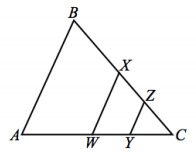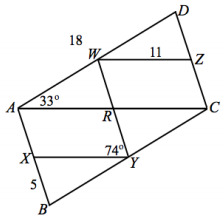PSA2PWM - Problem Sets---Geometry, M1 Lesson 29 (G.CO.C.9, G.CO.C.10)
Part A)

Use your knowledge of triangle congruence criteria to write proofs for each of the following problem.is a midsegment of △ABC, andis a midsegment of △CWXBX=AW.What can you conclude about ∠A and ∠B? Explain why.

Modified from EngageNY ©Great Minds Disclaimer

Part B)

What is the relationship of the lengths ofand?

Fill in the blank:

YZ = __ AB

Type your answer below as a number (example: 5, 3.1, 4 1/2, or 3/2):
Part A)

Use your knowledge of triangle congruence criteria to write proofs for the following problem.andWXY, and Z are the midpoints of, and, respectively. AD=18, WZ=11, and BX=5. m∠WAC=33°, m∠XBY=73°, m∠RYX=74°, m∠DCA=74°.m∠DZW=_______

Modified from EngageNY ©Great Minds Disclaimer

Type your answer below as a number (example: 5, 3.1, 4 1/2, or 3/2):
Part B)

Perimeter of ABYW = _______

Type your answer below as a number (example: 5, 3.1, 4 1/2, or 3/2):
Part C)

Perimeter of ABCD = _______

Type your answer below as a number (example: 5, 3.1, 4 1/2, or 3/2):
Part D)

m∠WAX=_______

Type your answer below as a number (example: 5, 3.1, 4 1/2, or 3/2):
Part E)

m∠B=_______

Type your answer below as a number (example: 5, 3.1, 4 1/2, or 3/2):
Part F)

m∠YCZ=_______

Type your answer below as a number (example: 5, 3.1, 4 1/2, or 3/2):
Part G)

m∠D=_______